Teaching support from the UK’s largest provider of in-school maths tuitionone to one lessonsschools supported

Built by teachers for teachers

In-school online one to one maths tuition developed by maths teachers and pedagogy experts

Find out more

Hundreds of FREE online maths resources!

Daily activities, ready-to-go lesson slides, SATs revision packs, video CPD and more!Explore all resources
Maths Mastery

Built by teachers for teachers

In-school online one to one maths tuition developed by maths teachers and pedagogy experts

Find out more

# White Rose Maths Year 6: What Students Learn And The Resources To Support Them

Here we look at the White Rose Maths Year 6 scheme of learning, the topics it covers across the year and provide lots of sample questions and White Rose Maths resources for those following White Rose in Year 6.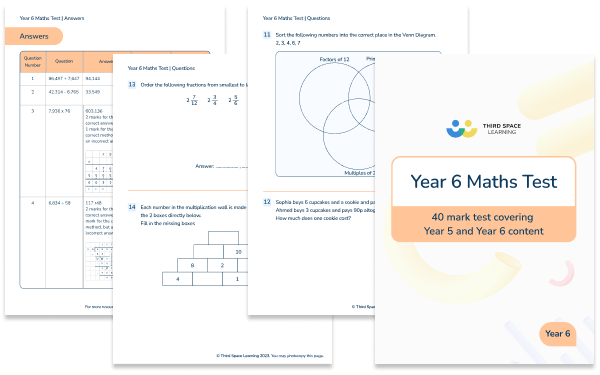Year 6 Maths Test

Download this free Year 6 maths test to check that your class is on track with their Year 6 maths curriculum.Download Free Now!

The White Rose Maths scheme of learning follows the national curriculum with a mastery approach to maths with a range of fluency, reasoning and problem solving elements. They also encourage concrete, pictorial and abstract representations to develop deep understanding of mathematical concepts.

It is thought that approximately 70% of schools in the UK follow the White Rose Maths scheme and this is reflected in the thousands of young mathematicians Third Space Learning tutors every week. Our tutors are highly skilled maths and pedagogy experts, well trained in the language, representations and approach used by White Rose. They are therefore able to deliver a one to one lesson that feels familiar to students who are also used to White Rose.

Throughout this article you’ll see example maths lesson slides and example questions from Third Space Learning’s Year 6 maths curriculum that exemplify best practice teaching to the White Rose Maths Year 6 scheme.

We’ve also provided lots of links to White Rose Maths Year 6 resources that are suitable for use for each topic.In addition to the links provided, Third Space Learning also offers libraries for White Rose Maths Year 1, White Rose Maths Year 2, White Rose Maths Year 3, White Rose Maths Year 4, and White Rose Maths Year 5

## About the White Rose Maths Year 6 Scheme of Learning

The primary school White Rose Maths scheme of learning has gone through several iterations and versions up to the current version 3. The scheme of learning SOL is designed as guidance and it is not necessarily intended for each small step to take one lesson. As with all teaching, it is important that teachers adapt this SOL for their class/ pupils.

### Topics covered in Year 6 White Rose Maths scheme of learning work

The White Rose Maths Year 6 SOL outlines which topics are taught, and when, throughout the autumn, spring and summer terms of Year 6.

The breakdown for Version 3 of the scheme of learning is as follows

#### Year 6 autumn term

• Place value
• Addition, subtraction, multiplication and division
• Fractions A
• Fractions B
• Converting units of measurement

#### Year 6 spring term

• Ratio
• Algebra
• Decimals
• Fractions, decimals and percentages
• Area, perimeter and volume
• Statistics

#### Year 6 summer term

• Shape
• Position and direction
• Themed projects, consolidation and problem solving

​​Third Space Learning has created several collections of White Rose Maths aligned resources for all primary year groups from early years through to Year 6. These resources can provide a different perspective on a topic and so help pupils spend the time needed to fully embed a concept.

Here is a summary of the different collections available for schools following Year 6 White Rose Maths, many of them are free resources and some are premium resources, all can be downloaded from our maths hub. Links to the topic specific versions are provided within each termly breakdown below:

These ready-to-go lesson slides come in the form of editable powerpoints with related worksheets that are designed for everyday teaching. The lesson slides cover both version 2 of the White Rose scheme of learning (in blue) and version 3 of the SOL. They also include support slides that can be used to support pupils who need a little extra  pre/ post teaching to support their understanding.

Code Crackers

Our Code Crackers resources are aligned to the version 2 SOL. These are summative resources designed as a retrieval activity for the end of the place value block.

Maths Code Crackers Year 6

Pre and Post Diagnostic Questions

These tests can be used before starting the place value block to assess gaps, and after teaching the place value block to identify progress.

Diagnostic Assessments Year 6

Worked Examples

Pupils check over completed questions to identify errors (or identify correct answers). Pupils are encouraged to explain the errors they find, not just ‘mark’ work. These worked examples help to solidify understanding by getting the pupil to discuss errors and how to avoid them.

Year 6 Worked Examples

Rapid Reasoning

These reasoning problems go alongside our Fluent in Five resource. One question each week has a model answer so teachers can understand what the question is asking and how to solve it.

Rapid Reasoning Year 6

All Kinds of Word Problems

Test your class’ problem solving skills with these place value word problems.

All Kinds of Word Problems Year 6

## White Rose Maths Year 6 autumn term

In the autumn block, Year 6 focuses on place value; addition, subtraction, multiplication & division; fractions and converting units of measurement.

### Place value Year 6

As  with all year groups, place value is the first block to be covered in the Autumn term. By Year 6, this is only a short unit of work; reading and writing numbers to 10,000,000 and exploring powers of 10.

#### Example year 6 place value questions

1. Write the following number in digits: twenty seven million, three hundred and forty eight thousand, five hundred and 6.

2. Identify the value of each underlined digit

a) 73,640,328

b) 25,604,713

c) 17,394,801

a) 3 million

b) 600,000

c) 90,000

### Addition, subtraction, multiplication and division Year 6

Addition, subtraction, multiplication and division is the largest block of Year 6. Unlike the other year groups, the four operations are all covered in one block, rather than being separated into two separate blocks. By this stage, pupils are expected to be confident using formal written methods for addition and subtraction. Focus is on securing confidence with long multiplication and division, and applying this knowledge to solving more complex multi-step problems. Learners also build on their understanding of factors, multiples, prime, square and cube numbers.

#### Example year 6 addition, subtraction, multiplication and division questions

1. 196,877 ÷ 37 =

2. Which number is a factor of 24, but not a multiple of 8?

a) 8

b) 7

c) 10

d) 12

### Fractions Year 6

Fractions in Year 6, are also split into two blocks, with both Block A and Block B being covered in the Autumn term.

Fractions A in Year 6

Pupils continue to develop their understanding of equivalent fractions in Year  6; utilising this knowledge to be able to compare and order fractions, and add and subtract fractions and mixed numbers with different denominators.

#### Example year 6 fractions A questions

1. Order the following fractions from largest to smallest

\frac{1}{2}, \frac{2}{3}, \frac{9}{12}, \frac{5}{6}

2. 4\frac{3}{4} + 5\frac{4}{12}

### Fractions B Year 6

In this second fractions block of Year 6, pupils build on the concepts introduced in Year 5; including multiplying and dividing fractions. They also continue to focus on calculating fractions of an amount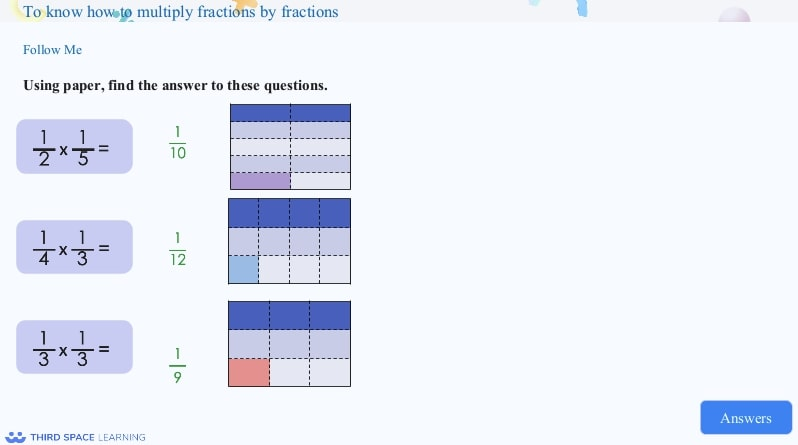Pupils are encouraged to explore multiplying two fractions using paper to help them understand how they get the answer.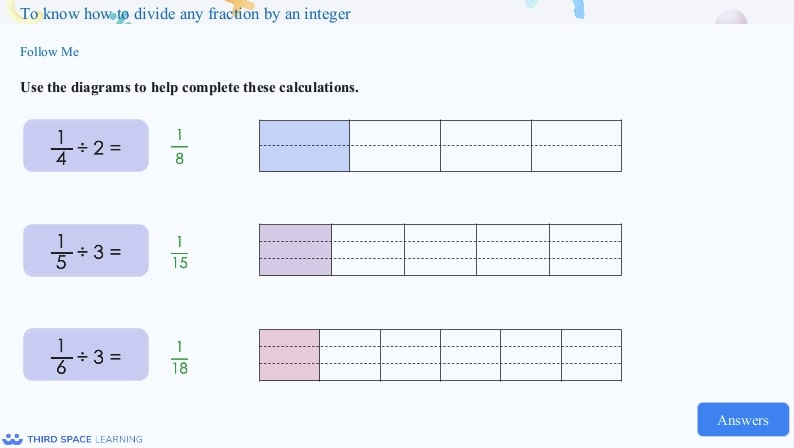When pupils learn how to divide a fraction by an integer, they are encouraged to represent the problem pictorially to help understand how the answer is found.

#### Example year 6 fractions B questions

1. 2\frac{1}{2} × 3\frac{1}{4} =

2. What is \frac{7}{8} of 336

### Converting units Year 6

Converting units is a short topic at the end of the autumn term. In this block, pupils build on their understanding of converting metric measures and are introduced to converting between metric and imperial measures (For example, between miles and kilometres).

#### Example year 6 converting units questions

1. Write 7,532m as km

2. If 1 mile = 1.6km, how far in kilometres is 8 miles?

## White Rose Maths Year 6 spring term

In the spring term term, Year 6 focuses on ratio; algebra; fractions, decimals & percentages; area, perimeter & volume and statistics

### Ratio Year 6

Pupils are introduced to ratio for the first time in Year 6. In this block pupils learn to use ratio language and the symbol. They also look at the connection between ratio and fractions and scale drawings.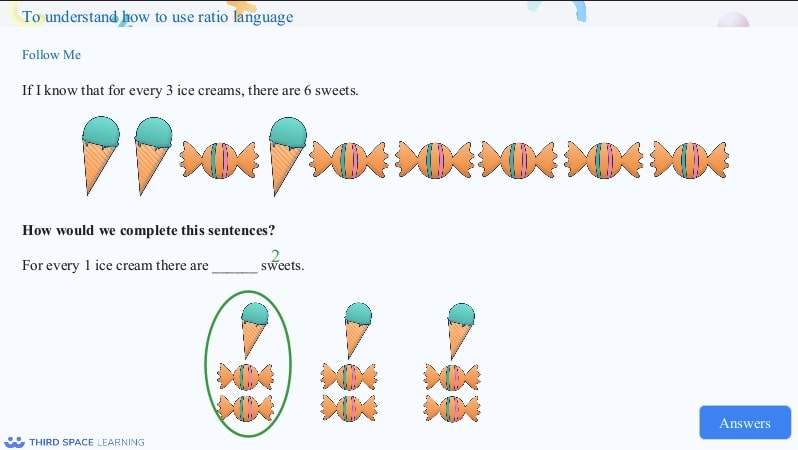Pupils are introduced to ratio in Year 6. They explore the language of ratio and use images to describe ratios.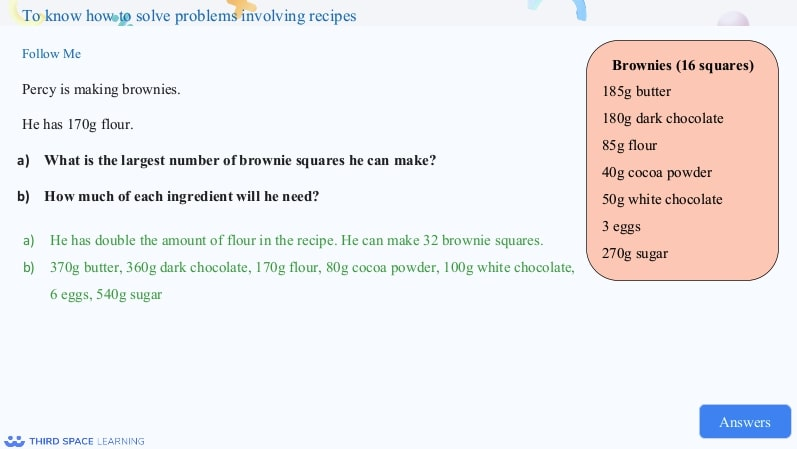Once pupils are familiar with ratio and proportion they explore problems, including problems involving recipes.

#### Example year 6 ratio questions

1. Gemma is making a necklace. The ratio of purple beads to blue beads she uses is 3:1. If she uses 5 blue beads, how many purple beads does she use?

2. The ratio of counters was 4:1 (red:yellow). If there were 20 counters altogether. How many red and yellow counters were there?

Answer: 16 red and 4 yellow

### Algebra Year 6

Year 6 is the first time pupils are introduced to algebra. Students begin the block investigating 1-step and 2-step function machines, followed by forming expressions, formulae and equations. This progresses to solving 1-step, then 2-step equations.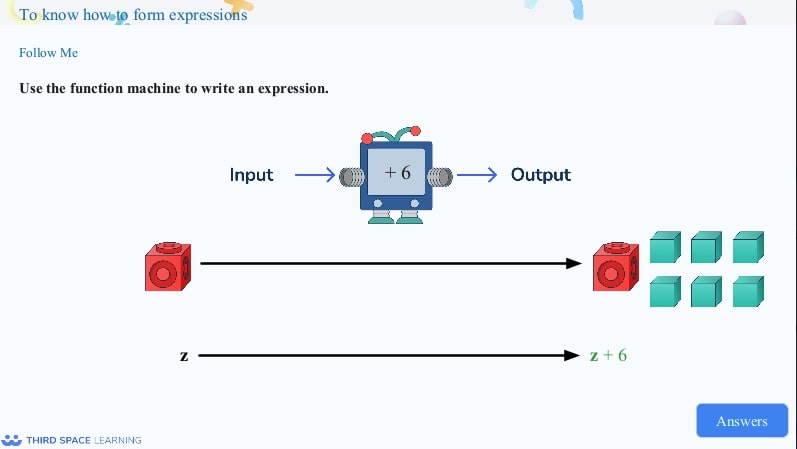Once pupils are familiar with function machines, they use function machines to write an expression. This is expressed using concrete resources or pictorial representations before moving on to abstract concepts.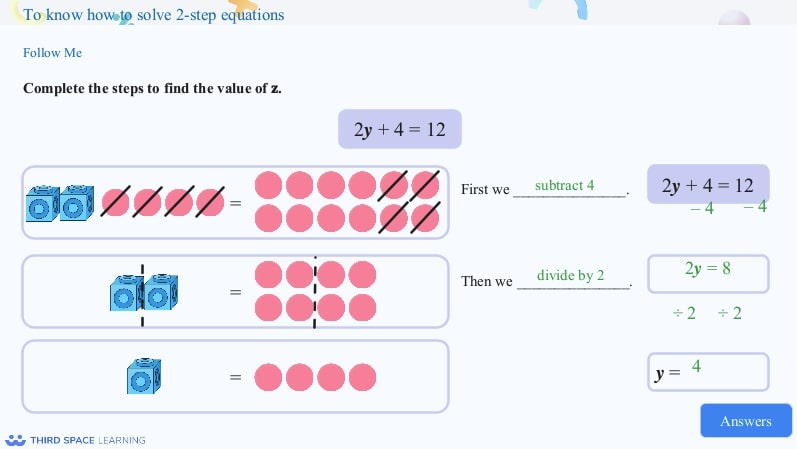Pupils use concrete or pictorial representations to solve 1-step and 2-step equations.

#### Example year 6 algebra questions

1. An x was input into the following function machine. What would be the output expression?

2. Solve the following equation: 2x + 2 = 12

### Decimals Year 6

In Year 6, pupils focus on decimals in two blocks. Firstly as an independent unit, followed by a unit covering fractions, decimals and percentages. In this first block, understanding of place value within a decimal number is reinforced, followed by rounding and adding & subtracting decimals and finally building on knowledge from year 5 to multiply and divide by 10, 100 and 1000.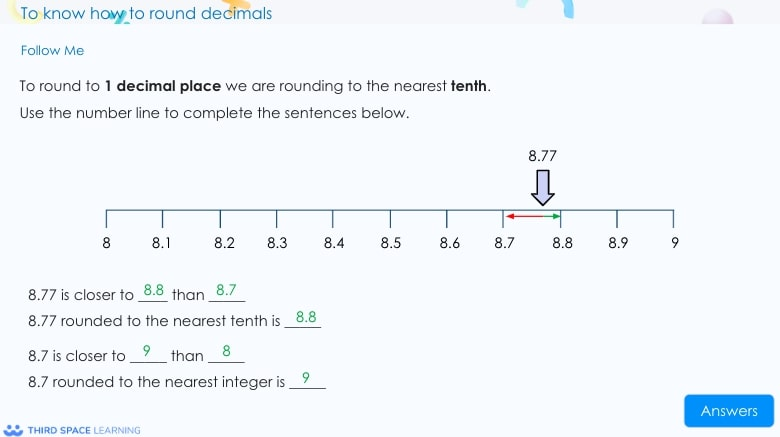Pupils look at rounding decimals to the nearest whole or the nearest tenth. They are encouraged to use number lines to help understand how to round the numbers.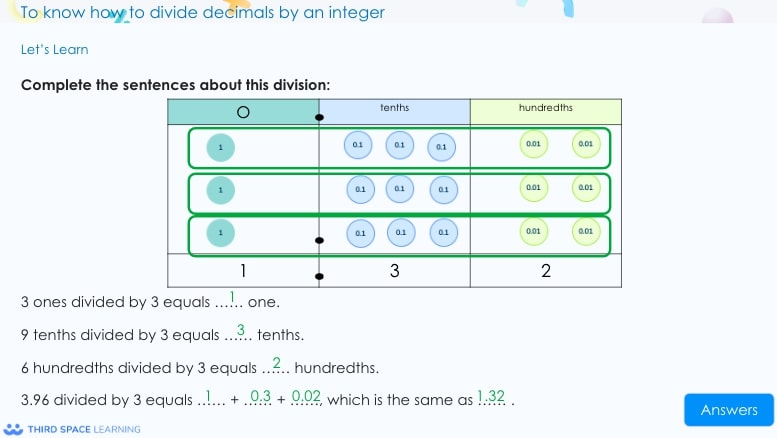Pupils use concrete resources to help them understand how to divide decimals by an integer.

#### Example year 6 decimals questions

1. Round 3.765 to 2 decimal places

2. Divide 32.7 by 10, 100 and 100

32.7 ÷ 10 = 3.27

32.74 ÷ 100 =0.327

32.74 ÷ 1000 =0.0327

### Fractions, decimals and percentages Year 6

In this block, pupils build upon the concepts learnt in Year 5; exploring and using equivalence between fractions, decimals and percentages. At this stage, students are also introduced to the concept of calculating a percentage of an amount. Initially pupils are introduced to  one-step problems, such as finding 10% of an amount. They then progress to solving multi-step problems, such as calculating 37% of an amount.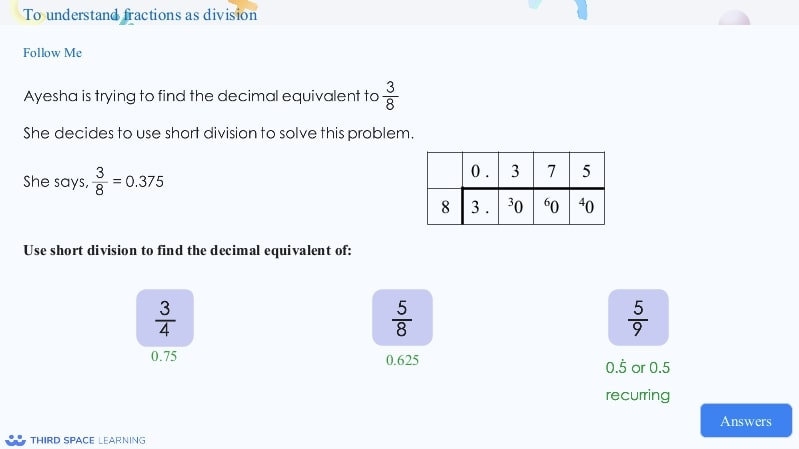Pupils look at how to convert fractions to decimals using different methods, such as using short division.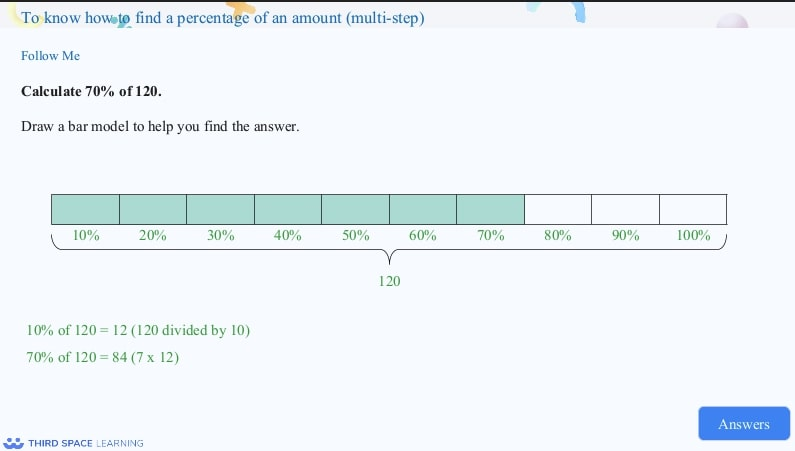Pupils should understand what a percentage is by Year 6. They can then go on to find percentages of amounts (both in multiples of 10 and multiples of 5).

#### Example year 6 fractions, decimals and percentages questions

1. Write \frac{7}{25} as a decimal and a percentage

Answer: \frac{28}{100} = 0.28 = 28%

2. Use the < > or = symbol for each question below:

\frac{4}{10}   ______  45%

\frac{3}{4} ________ 70%

\frac{6}{20} ______  60%

\frac{4}{10}   < 45%

\frac{3}{4}  > 70%

\frac{6}{20} <  60%

### Area, perimeter and volume Year 6

In Year 6, pupils explore how to calculate the area of different shapes; including triangles and parallelograms. They also build on their knowledge of volume from Year 5; calculating, estimating and comparing volume of cubes and cuboids, using standard units, including cubic centimetres and cubic metres.

#### Example year 6 area, perimeter and volume questions

1. Calculate the volume of the cuboid below

2. Draw 2 different cuboids with a volume of 60cm ^{3}

Answer: 2 x 5 x 6 and 3 x 4 x 5

### Statistics Year 6

In Year 6, pupils continue to focus on drawing and interpreting line graphs. They are also introduced to pie charts for the first time, learning how to interpret and construct them. Students also learn how to calculate and interpret the mean as an average.

#### Example year 6 statistics questions

1. A man walks up a hill. It takes him 15 minutes to reach 800m. He then stops for 10 minutes before walking back down. It takes him 10 minutes to reach the bottom of the hill. Plot the graph to show what happens.

## White Rose Maths Year 6 summer term

In the summer term, Year 6 focuses on shape; position & direction, followed by themed projects, consolidation and problem solving.

### Shape Year 6

Pupils build on their understanding of shape and properties of shape; drawing 2D shapes, using given dimensions. Students also learn to recognise, describe and build simple 3D shapes, including making nets. In Year 6, there is a significant focus on angles, with pupils learning how to calculate a range of angles, including: vertically opposite angles; angles in triangles, quadrilaterals and other polygons.

#### Example year 6 shape questions

1. Calculate the size of angle a below:

2. A triangle has the angles: 45° and 65°. Calculate what the 3rd angle will be:

### Position and direction Year 6

In Year 4 and 5, pupils learn to plot coordinates in the first quadrant. In Year 6, pupils progress to reading and plotting points in all four quadrants and solving problems with coordinates. Students also draw and translate simple shapes on the coordinate plane, and reflect them in the axes.

#### Example year 6 position and direction questions

1. On the grid below, plot the following coordinates. What shape do they make?

(4,3) (-2,3) (-2, -2) (3,-2)

They make a square

#### Looking for more year 6 maths resources and guidance?

Do you have pupils who need extra support in maths?
Every week Third Space Learning’s maths specialist tutors support thousands of pupils across hundreds of schools with weekly online 1-to-1 lessons and maths interventions designed to plug gaps and boost progress.

Since 2013 we’ve helped over 150,000 primary and secondary school pupils become more confident, able mathematicians. Learn more or request a personalised quote for your school to speak to us about your school’s needs and how we can help.

Subsidised one to one maths tutoring from the UK’s most affordable DfE-approved one to one tutoring provider.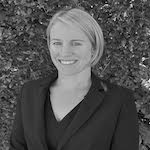##### Emma Johnson
Emma is a former Deputy Head Teacher, with 12 years' experience leading primary maths. Over the past 18 years, she has worked in primary schools in the UK and internationally, in Qatar. She now runs a tutoring company and writes resources and blogs for Third Space Learning, She is also the creator of the YouTube channel Maths4Kids with her daughter, Amber.

Related Articles

x#### Year 6 Maths Test

Download this free Year 6 maths test to check that your class is on track with their Year 6 maths curriculum.

#### Year 6 Maths TestDownload this free Year 6 maths test to check that your class is on track with their Year 6 maths curriculum.